# 2.2 - One-Way ANOVA Sums of Squares, Mean Squares, and F-test

by

The previous discussion showed two ways of estimating the model but still hasn't addressed how to assess evidence related to whether the observed differences in the means among the groups is "real". In this section, we develop what is called the ANOVA F-test that provides a method of aggregating the differences among the means of 2 or more groups and testing our null hypothesis of no difference in the means vs the alternative. In order to develop the test, some additional notation needs to be defined. The sample size in each group is denoted nj and the total sample size is N=Σnj = n1+n2+...+nJ where Σ (capital sigma) means "add up over whatever follows". An estimated residual (eij) is the difference between an observation, γij, and the model estimate, γ̂ij=μ̂j, for that observation, γij - γ̂ij=eij. It is basically what is left over that the mean part of the model (μ̂j) does not explain and is our window into how "good" the model might be.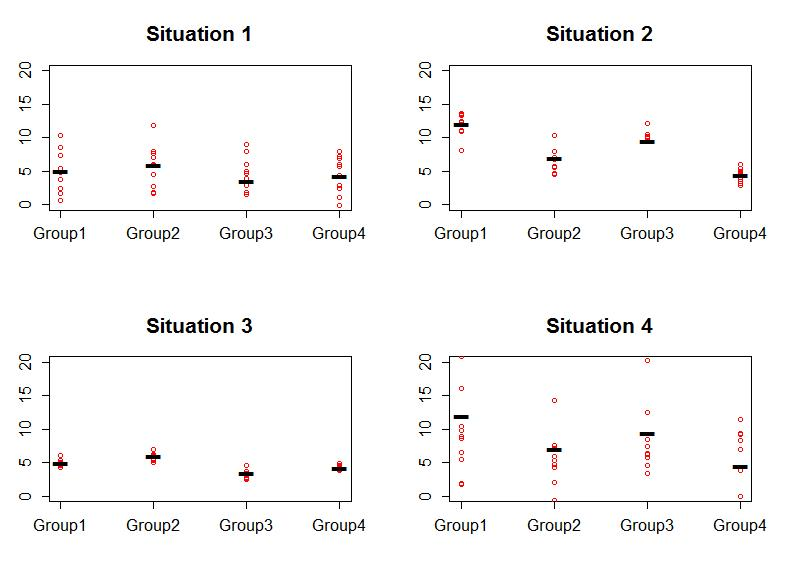Figure 2-3: Demonstration of different amount of difference in means relative to variability.

Consider the four different fake results for a situation with four groups in Figure 2-3. In Situation 1, it looks like there is little evidence for a difference in the means and in Situation 2, it looks fairly clear that there is a difference in the group means. Why? It is because the variation in the means looks "clear" relative to the variation around the means. Consider alternate versions of each result in Situations 3 and 4 and how much evidence there appears to be for same sizes of differences in the means. In the plots, there are two sources of variability in the responses - how much the group means vary across the groups and how much variability there is around the means in each group. So we need a test statistic to help us make some sort of comparison of the groups and to account for the amount of variability present around the means. The statistic is called the ANOVA F-statistic. It is developed using sums of squares which are measures of total variation like used in the numerator of the standard deviationthat took all the observations, subtracted the mean, squared the differences, and then added up the results over all the observations to generate a measure of total variability. With multiple groups, we will focus on decomposing that total variability (Total Sums of Squares) into variability among the means (we'll call this Explanatory Variable A's Sums of Squares) and variability in the residuals or errors (Error Sums of Squares). We define each of these quantities in the One-Way ANOVA situation as follows:

• ⚪ SSTotal = Total Sums of Squares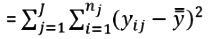• ■ By summing over all nj observations in each group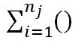and then adding those results up across the groups, we accumulate the variation across all N observations.
• ■ Total variation is assessed by squaring the deviations of the responses around the overall or grand mean (γ̿, the estimated mean for all the observations and available from the mean-only model).
• ■ Note: this is the residual variation if the null model is used, so there is no further decomposition possible for that model.
• ■ This is also equivalent to the numerator of the sample variance which is what you get when you ignore the information on the potential differences in the groups.
• ⚪ SSA = Explanatory Variable A's Sums of Squares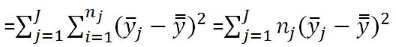• ■ Variation in the group means around the grand mean based on explanatory variable A.
• ■ Also called sums of squares for the treatment, regression, or model.
• ⚪ SSE = Error (Residual) Sums of Squares• ■ Variation in the responses around the group means.
• ■ Also called the sums of squares for the residuals.

The possibly surprising result given the mass of notation just presented is that the total sums of squares is ALWAYS equal to the sum of explanatory variable A's sum of squares and the error sums of squares, SSTotal = SSA + SSE. This equality means that if the SSA goes up, then the SSE must go down if SSTotal remains the same. This result is called the sums of squares decomposition formula. We use these results to build our test statistic and organize this information in what is called an ANOVA table. The ANOVA table is generated using the anova function applied to the reference-coded model:

> lm2 <- lm(Years~Attr,data=MockJuryR)

> anova(lm2)

Analysis of Variance Table

Response: Years

 Df Sum Sq Mean Sq F value Pr(>F) Attr 2 70.94 35.469 2.77 0.067 . Residuals 111 1421.32 12.805

Note that the ANOVA table has a row labelled Attr, which contains information for the grouping variable (we'll generally refer to this as explanatory variable A but here it is the picture group that was randomly assigned), and a row labelled Residuals, which is synonymous with "Error". The SS are available in the Sum Sq column. It doesn't show a row for "Total" but the SSTotal =SSA+SSE = 1492.26.

> 70.94+1421.32

 1492.26

It may be easiest to understand the sums of squares decomposition by connecting it to our permutation ideas. In a permutation situation, the total variation (SSTotal) cannot change - it is the same responses varying around the grand mean. However, the amount of variation attributed to variation among the means and in the residuals can change if we change which observations go with which group. In Figure 2-4, the means and 95% confidence intervals are displayed for the three treatment levels. In panel (a), the results for the original data set (a) are presented including sums of squares. Three permuted versions of the data set are summarized in panels (b), (c), and (d). The SSA is 70.9 in the real data set and between 6.6 and 11 in the permuted data sets. If you had to pick among the plots for the one with the most evidence of a difference in the means, you hopefully would pick panel (a). This visual "unusualness" suggests that this observed result is unusual relative to the possibilities under permutations, which are, again, the possibilities tied to having the null hypothesis being true. But note that the differences are not that great between these permuted data sets and the real one.

One way to think about SSA is that it is a function that converts the variation in the group means into a single value. This makes it a reasonable test statistic in a permutation testing context. By comparing the observed SSA=70.9 to the permutation results of 6.7, 6.6, and 11 we see that the observed result is much more extreme than the three alternate versions. In contrast to our previous test statistics where positive and negative differences were possible, SSA is always positive with a value of 0 corresponding to no variation in the means. The larger the SSA, the more variation there was in the means. The permutation p-value for the alternative hypothesis of some (not of greater or less than!) difference in the true means of the groups will involve counting the number of permuted SSA* results that are larger than what we observed.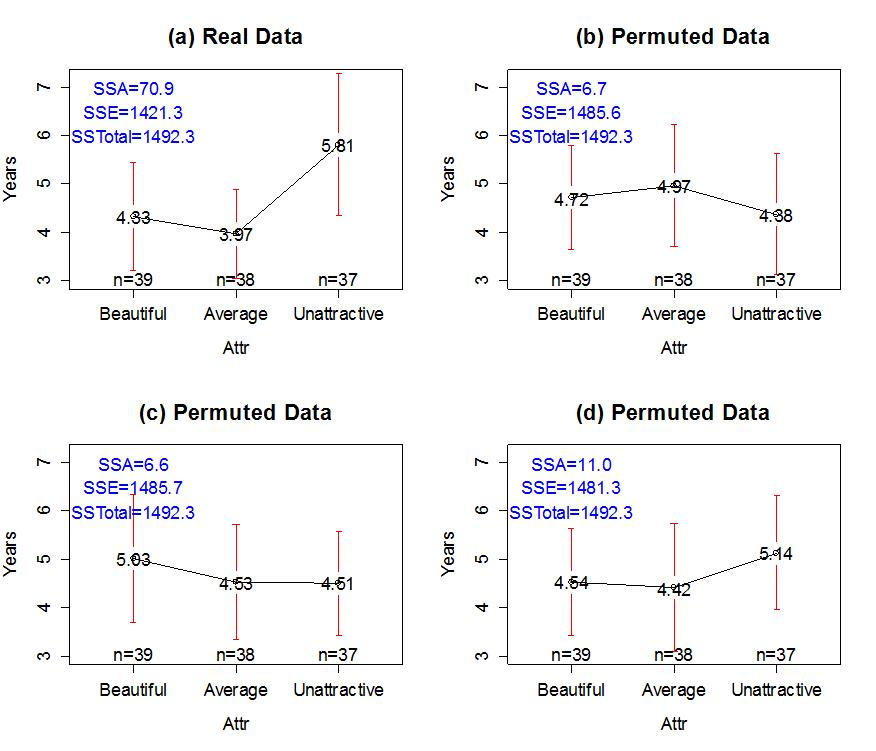Figure 2-4: Plot of means and 95% confidence intervals for the three groups for the real data (a) and three different permtutations of the treatment labels to the same responses in (b), (c), and (d).

To do a permutation test, we need to be able to calculate and extract the SSA value. In the ANOVA table, it is in the first row and is the second number and we can use the [,] referencing to extract that number from the ANOVA table that anova produces (anova(lm(Years~Attr,data=MockJury))[1,2]). We'll store the observed value of SSA is Tobs:

> Tobs <- anova(lm(Years~Attr,data=MockJury))[1,2]; Tobs

 70.93836

The following code performs the permutations using the shuffle function and then makes a plot of the resulting permutation distribution:

> B<-1000

> Tstar<-matrix(NA,nrow=B)

> for (b in (1:B)){

+ Tstar[b]<-anova(lm(Years~shuffle(Attr),data=MockJury))[1,2]

+ }

> hist(Tstar,labels=T)

> abline(v=Tobs,col="red",lwd=3)

> plot(density(Tstar),main="Density curve of Tstar")

> abline(v=Tobs,col="red",lwd=3)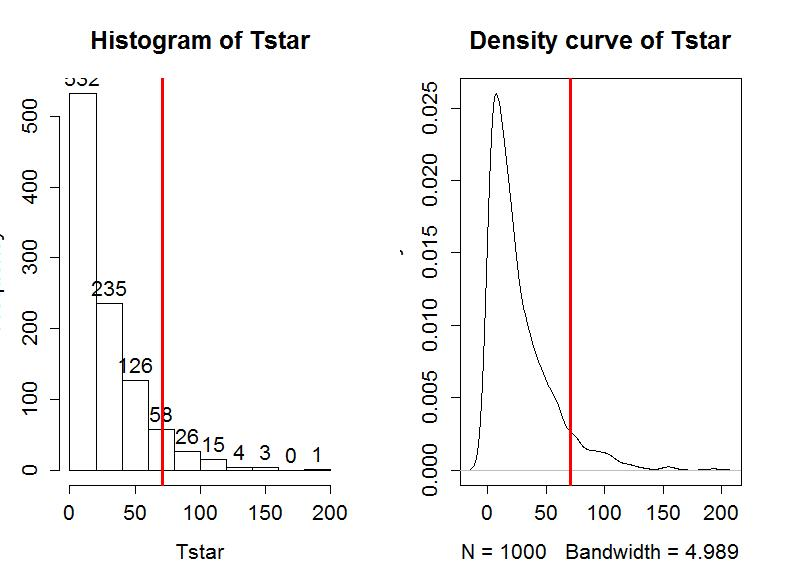Figure 2-5: Permutation distributions of SSA with the observed value of SSA (bold, vertical line).

The right-skewed distribution (Figure 2-5) contains the distribution of SSA*'s under permutations (where all the groups are assumed to be equivalent under the null hypothesis). While the observed result is larger than many SSA*'s, there are also many results that are much larger than observed that showed up when doing permutations. The proportion of permuted results that exceed the observed value is found using pdata as before, except only for the area to the right of the observed result. We know that Tobs will always be positive so no absolute values are required now.

> pdata(Tobs,Tstar,lower.tail=F)

 0.071

This provides a permutation-based p-value of 0.071 and suggests marginal evidence against the null hypothesis of no difference in the true means. We would interpret this as saying that there is a 7.1% chance of getting a SSA as large or larger than we observed, given that the null hypothesis is true.

It ends up that some nice parametric statistical results are available (if our assumptions are met) for the ratio of estimated variances, which are called Mean Squares. To turn sums of squares into mean square (variance) estimates, we divide the sums of squares by the amount of free information available. For example, remember the typical variance estimator introductory statistics,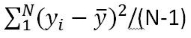, where we "lose" one piece of information to estimate the mean and there are N deviations around the single mean so we divide by N-1. Now consider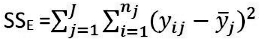which still has N deviations but it varies around the J means, so the Mean Square Error = MSE = SSE/(N-J). Basically, we lose J pieces of information in this calculation because we have to estimate J means. The sums of squares for explanatory variable A is harder to see in the formula, but the same reasoning can be used to understand the denominator for forming the Mean Square for variable A or MSA: there are J means that vary around the grand mean so MSA = SSA/(J-1). In summary, the two mean squares are simply:

• ■ MSA = SSA/(J-1), which estimates the variance of the group means around the grand mean.
• ■ MSError = SSError/(N-J), which estimates the variation of the errors around the group means.

These results are put together using a ratio to define the ANOVA F-statistic (also called the F-ratio) as

F=MSA/MSError.

This statistic is close to 1 if the variability in the means is "similar" to the variability in the residuals and would lead to no evidence being found of a difference in the means. If the MSA is much larger than the MSE, the F-statistic will provide evidence against the null hypothesis. The "size" of the F-statistic is formalized by finding the p-value. The F-statistic, if assumptions discussed below are met and we assume the null hypothesis is true, follows an F-distribution. The F-distribution is a right-skewed distribution whose shape is defined by what are called the numerator degrees of freedom (J-1) and the denominator degrees of freedom (N-J). These names correspond to the values that we used to calculate the mean squares and where in the F-ratio each mean square was used; F-distributions are denoted by their degrees of freedom using the convention of F(numerator df, denominator df). Some examples of different F-distributions are displayed for you in Figure 2-6.

The characteristics of the F-distribution can be summarized as:

• ⚪ Right skewed,
• ⚪ Nonzero probabilities for values greater than 0,
• ⚪ Shape changes depending on the numerator and denominator DF, and
• ⚪ Always use the right-tailed area for p-values.

Now we are ready to see an ANOVA table when we know about all its components. Note the general format of the ANOVA table is22:

 Source DF Sums of Squares Mean Squares F-ratio P-value Variable A J-1 SSA MSA = SSA/(J-1) F=MSA/MSE Right tail of F(J-1,N-J) Residuals N-J SSE MSE =SSE/(N-J) Total N-1 SSTotal

The table is oriented to help you reconstruct the F-ratio from each of its components. The output from R is similar although it does not provide the last row. The R version of the table for the type of picture effect (Attr) with J=3 levels and N=114 observations, repeated from above, is:

> anova(lm2)

Analysis of Variance Table

Response: Years

 Df Sum Sq Mean Sq F value Pr(>F) Attr 2 70.94 35.469 2.77 0.067 . Residuals 111 1421.32 12.805

The p-value from the F-distribution is 0.067. We can verify this result using the observed F-statistic of 2.77 (which came from the ratio of the means squares: 35.47/12.8) which follows an F(2,111) if the null hypothesis is true and other assumptions are met. Using the pf function provides us with areas in the specified F-distribution with the df1 provided to the function as the numerator DF and df2 as the denominator and lower.tail=F reflecting our desire for a right tailed area.

> pf(2.77,df1=2,df2=111,lower.tail=F)

 0.06699803

The result from the F-distribution using this parametric procedure is similar to the p-value obtained using permutations with the test statistic of the SSA, which was 0.071. The F-statistic obviously is another potential test statistic to use as a test statistic in a permutation approach. We should check that we get similar results from it with permutations as we did from using SSA as a test statistic. The following code generates the permutation distribution for the F-statistic (Figure 2-7) and assesses how unusual the observed F-statistic of 2.77 was in this permutation distribution. The only change in the code involves moving from extracting SSA to extracting the F-ratio which is in the 4th column of the anova output:

> anova(lm(Years~Attr,data=MockJury))[1,4]

 2.770024

> Tobs <- anova(lm(Years~Attr,data=MockJury))[1,4]; Tobs

 2.770024

> B<-1000

> Tstar<-matrix(NA,nrow=B)

> for (b in (1:B)){

+ Tstar[b]<-anova(lm(Years~shuffle(Attr),data=MockJury))[1,4]

+ }

> hist(Tstar,labels=T)

> abline(v=Tobs,col="red",lwd=3)

> plot(density(Tstar),main="Density curve of Tstar")

> abline(v=Tobs,col="red",lwd=3)

> pdata(Tobs,Tstar,lower.tail=F)

 0.064Figure 2-7: Permutation distribution of the F-statistic with bold, vertical line for observed value of test statistic of 2.77.

The permutation-based p-value is 0.064 which, again, matches the other results closely. The first conclusion is that using a test statistic of the F-statistic or the SSA provide similar permutation results. However, we tend to favor using the F-statistic because it is more commonly used in reporting ANOVA results, not because it is any better in a permutation context.

It is also interesting to compare the permutation distribution for the F-statistic and the parametric F(2,111) distribution (Figure 2-8). They do not match perfectly but are quite similar. Some the differences around 0 are due to the behavior of the method used to create the density curve and are not really a problem for the methods. This explains why both methods give similar results. In some situations, the correspondence will not be quite to close.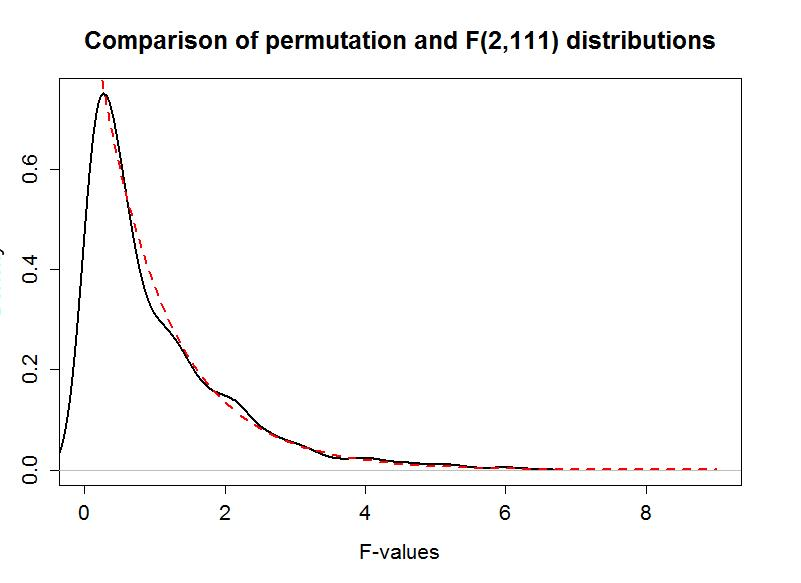Figure 2-8: Comparison of F(2,111) (dashed line) and permutation distribution (solid line).

So how can we rectify this result (p-value≈0.06) and the Chapter 1 result that detected a difference between Average and Unattractive with a p-value≈0.03? I selected the two groups to compare in Chapter 1 because they were furthest apart. "Cherry-picking" the comparison that is likely to be most different creates a false sense of the real situation and inflates the Type I error rate because of the selection. If the entire suite of comparisons are considered, this result may lose some of its luster. In other words, if we consider the suite of all pair-wise differences (and the tests) implicit in comparing all of them, we need stronger evidence in the most different pair than a p-value of 0.033 to suggest overall differences. The Beautiful and Average groups are not that different from each other so they do not contribute much to the overall F-test. In Section 2.5, we will revisit this topic and consider a method that is statistically valid for performing all possible pair-wise comparisons.

22Make sure you can work from left to right and up and down to fill in the ANOVA table given just the necessary information to determine the other components - there is always a question like this on the exam...